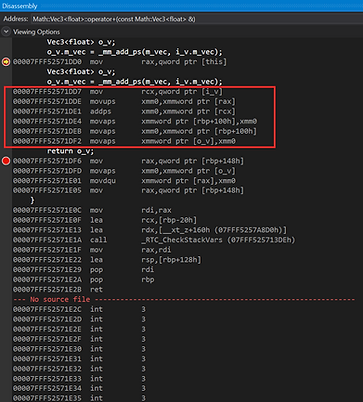##### Improving Vector and Matrix

My main goals for this project are the two things below

1. Make the vector and matrix class expandable and flexible.

2. Optimize the calculation process as much as possible in a given situation.

The first thing I did is to separate the math class as a different module. Since the code structure was a bit messy, I need to clean up and unify classes.After that, I changed my vector and matrix class to templates. so that there will be expandable for future implementations.The picture above is the result. I wanted to have intrinsic instructions for a specific type of vector for a calculation optimization purpose.  So I made the structure as followed. The system will decide and use whichever implementation is best according to the compiler type and CPU architecture.

I didn't know that full template specialization doesn't inherit any function from the primary template like how class inheritance does. So I had to rewrite all the operators for each specific template :(iMatrix.inliMatrix.SSE2.inl

Right now, it only has simple intrinsic functions with SSE2. but I will add more as the project goes.

I think I have achieved the first goal, "1. Make the vector and matrix class cleaner and flexible".

Not, let's check if the second goal "2. Optimize the vector calculation as much as possible in the given situation." is satisfied.I disassemble the codes for two different versions of the addition operator for float vectors. The left side is the normal version and the right one is the intrinsic version. The instruction number gets significantly decreased in the intrinsic version, meaning the second goal is also achieved.

These are the information and questions that I found interesting while I was working on this project.

What is the difference between typename and class keywords in template

Pass by value is optimal in primitive types

What is ivdep

Intel Intrinsics Guide

The codes I mainly took as references are three codes below.

1. cyCodeBase : http://www.cemyuksel.com/cyCodeBase/index.html

2. Example game engine source code using in my class: